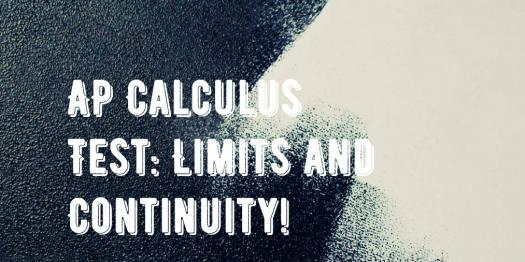# AP Calculus Test: Limits And Continuity!

10 Questions | Total Attempts: 197SettingsAP calculus test: Limits and Continuity is an integral part of high school and college mathematics. Engineering students and other students of mathematics, including physics and chemistry, also use it in solving problems. This quiz on Limits and Continuity focuses on an essential aspect of Calculus which test is important for anyone taking the Calculus Test.

• 1.
The mean value of a Sine wave over half a cycle is:
• A.

0.318 × maximum value

• B.

0.707 × maximum value

• C.

The peak value

• D.

0.637 × maximum value

• 2.
A triangle has sides a = 9.0 cm, b = 8.0 cm and c = 6.0 cm. Angle A is equal to:
• A.

82.42°

• B.

56.49°

• C.

78.58°

• D.

79.87°

• 3.
An arc of a circle of length 5.0 cm subtends an angle of 2 radians. The circumference of the circle is:
• A.

2.5 cm

• B.

10.0 cm

• C.

5.0 cm

• D.

15.7 cm

• 4.
Four engineers can complete a task in 5 hours. Assuming the rate of work remains constant, six engineers will complete the task in:
• A.

126h

• B.

4h 48min

• C.

3h 20min

• D.

7h 30min

• 5.
11 mm expressed as a percentage of 41 mm is:
• A.

2.68, correct to 3 significant figures

• B.

2.6, correct to 2 significant figures

• C.

26.83, correct to 2 decimal places

• D.

0.2682, correct to 4 decimal places

• 6.
The relationship between the temperature in degrees Fahrenheit (F) and the temperature in degrees Celsius (C) is given by: F = 9/5C + 32. 135◦F is equivalent to:
• A.

43◦C

• B.

57.2◦C

• C.

185.4◦C

• D.

184◦C

• 7.
The vertical displacement, s, of a prototype model in a tank is given by s = 40 sin 0.1t mm, where t is the time in seconds. The vertical velocity of the model, in mm/s, is:
• A.

− cos 0.1t

• B.

400 cos 0.1t

• C.

−400 cos 0.1t

• D.

4 cos 0.1t

• 8.
An alternating current is given by i = 4 sin 150t amperes, where t is the time in seconds. The rate of change of current at t = 0.025s is:
• A.

3.99A/s

• B.

−492.3A/s

• C.

−3.28A/s

• D.

598.7A/s

• 9.
The velocity of a car (in m/s) is related to time t seconds by the equation v = 4.5 + 18t − 4.5t 2. The maximum speed of the car, in km/h, is:
• A.

81

• B.

6.25

• C.

22.5

• D.

77

• 10.
A vehicle has a velocity v = (2 + 3t) m/s after t seconds. The distance travelled is equal to the area under the v/t graph. In the first 3 seconds the vehicle has travelled:
• A.

11m

• B.

33m

• C.

13.5m

• D.

19.5m

Related TopicsBack to top